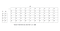# Learn Dynamic Programming by Solving Knapsack Problem in Java

“A lot of problems we face in life, be it business, finance, including logistics, container ship loading, aircraft loading -these are all knapsack problems,” — Carsten MurawskiPhoto by Jeremy Bishop on Unsplash

# What is Dynamic Programming?

In this article, I am going to discuss solving knapsack problems using dynamic programming. Dynamic Programming is a method for solving some types of problems in polynomial time.

1. Optimal Substructure Property

# What is Knapsack problem?💼

When given a set of items with a weight and a value, we have to determine the number of each item to include in a collection. The total weight is less than or equal to the given limit. And the total value of the items should be maximized.

# How Knapsack problem related to Dynamic Programming?

Typically, Dynamic Programming may solve all problems involving maximizing or minimizing specific quantities, counting problems that require counting the arrangements under certain conditions, or specific probability problems.

• Fractional Knapsack problem algorithm. In this type, we can break items to maximize the total value of the knapsack. The Greedy Strategy can solve this type.

# How to Solve the Knapsack problem?

Question:

There are given weights and values of n items, respectively, put these items in a knapsack of capacity W to get the maximum total value in the knapsack. Find out the maximum value subset of value[]. The sum of the weights of this subset is smaller than or equal to W. You cannot break an item. Either pick the complete item or don’t pick it (0–1 property).

weights ={ 3, 6,4, 5}
values = {2, 3, 6, 4}
W = 8 (Maximum weight that can be carried)
n = 4 ( no. of items )

So, our first step will be deciding a state for the problem after identifying that the problem is a Dynamic Programming problem.
As we know, Dynamic Programming is all about using calculated results to formulate the final result.
Our next step will be to find a relation between previous states to reach the current state. So we have to store the subproblems in a table and finally get the result.

## Steps to divide the problem into subproblems

`step1: Create a table with 0 - W values on the horizontal axis and 0-n values vertical axis. ( think the table as matrix m)step2: Arrange the given weights into ascending order.step3: You can fill out the table like the below formula to calculate the answer for each sub-problem.m[i,W] = max( m[i-1,W] , v[i]+ m[i-1 , W - W[i])`Figure 01: Table of sub problems

## Steps to find the solution

`step1: Set the pointer to the last row last column of the table( value = 8)step2: move the pointer upward until the value changed( Here, we have to go two cells upward) and select the weight in that row( Weight = 4)step3: Now the remaining weight is 4 (8-4). Move the pointer one cell up and go to the column where weight is 4 (value = 2)step4: Repeat step 2 and three until i =0`

# Conclusion

Here we arrived at the end of this article. So far, we learned How the 0/1 Knapsack problem solves in Dynamic Programing. I hope this article helped you to develop your knowledge about these concepts. Let’s meet with another interesting article soon.

## References

Knapsack problem — Wikipedia. https://en.wikipedia.org/wiki/Knapsack_problem

## Javarevisited

Medium’s largest Java publication, followed by 11300+ programmers. Follow to join our community.

Thanks to Trey Huffine

Written by

## Pulsara Sandeepa

Tech Enthusiast | Undergraduate | Blogger

## Javarevisited

A humble place to learn Java and Programming better.

Written by

## Pulsara Sandeepa

Tech Enthusiast | Undergraduate | Blogger## Javarevisited

A humble place to learn Java and Programming better.

## More From Medium

Medium is an open platform where 170 million readers come to find insightful and dynamic thinking. Here, expert and undiscovered voices alike dive into the heart of any topic and bring new ideas to the surface. Learn more

Follow the writers, publications, and topics that matter to you, and you’ll see them on your homepage and in your inbox. Explore

If you have a story to tell, knowledge to share, or a perspective to offer — welcome home. It’s easy and free to post your thinking on any topic. Write on Medium